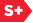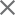### Proceedings Paper

Integration-free projection of a sampled signal on a multiresolution analysis ladder space via approximation theory
Author(s): Dumitru Mihai Ionescu; Mark A. Wickert; Jacqueline J. Hine
Format Member Price Non-Member Price
PDF \$17.00 \$21.00

Paper Abstract

Let Hs be the Sobolev space of exponent s >= 0. Let Vi, i (epsilon) Z,...Vi is contained in Vi-1 is contained in ..., be the ladder spaces of a multiresolution analysis (MRA) in L2(R) associated with a compactly supported scaling function (phi) (epsilon) H(sigma ) which verifies supp(phi) equals [ 0, L-1 ], L (epsilon) N, (sigma) > 1/2. Let f (epsilon) L2(R) be compactly supported with countable many discontinuity points and verifying suppf equals [ t1, t2 ], where 0 < (mu) (suppf) < (infinity) and, for i equals 1,2 and some integer m\$_0), ti equals ni(m0)2mo with ni(m0) (epsilon) Z. The goal is to approximate f by a function in Vm which approaches f in some sense as the scale becomes finer, reproduces the values of f at certain equally spaced, arbitrarily close points and is not necessarily the orthogonal projection of f on Vm. Such non-orthogonal projections can be used to start the pyramid algorithm or to approximate f, as an alternative which is better than using the samples themselves in place of the true inner products and faster than integrating for the inner products. The almost uniform convergence feature allows interactive control on the selection of an initial sampling rate that is capable of preserving details down to a desired resolution.

Paper Details

Date Published: 23 October 1996
PDF: 11 pages
Proc. SPIE 2825, Wavelet Applications in Signal and Image Processing IV, (23 October 1996); doi: 10.1117/12.255229
Show Author Affiliations

Published in SPIE Proceedings Vol. 2825:
Wavelet Applications in Signal and Image Processing IV
Michael A. Unser; Akram Aldroubi; Andrew F. Laine, Editor(s)